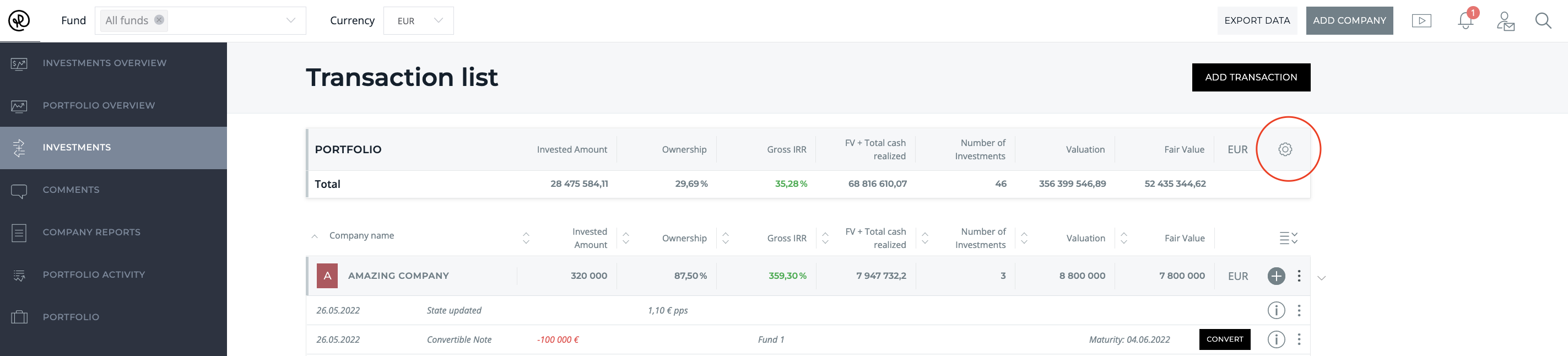# How does Rundit generate investment metrics?

## Learn how you could use Rundit to generate investment metrics, displayed both at company and fund level

From the Investments page, you are able to view company and fund level investment metrics. Click the Settings icon to select which metrics you'd like to be displayed.• Invested amount: The sum of all the investments
• Ownership: Calculates your ownership based on total number of shares in the company and shares owned by the fund.
• Gross IRR (Gross internal rate of return): Uses the same calculation as XIRR in Excel. It takes all transactions with dates and calculates the value. As a final transaction it uses current fair value (if you would exit today, what would be IRR).
• Multiple (Gross TVPI): (Fair value + total cash realized) / total invested amount
• ROI (Return on investment): (Fair value + total cash realized - total invested amount) / total invested amount x 100.
• Total cash realized: All realizations (Loan repayments including interest at the moment, dividends, other realizations, exits, insolvency).
• Fair value: Default calculation takes value of unrealized equity based on the latest price per share, face value of unconverted notes, and face value of other investments.
• Fair value + Total cash realized: The sum of fair value and total cash realized
• Number of investments: The number of investments (minus transactions). Doesn't take into account realizations or state updates.
• Valuation: Total number of shares outstanding x Price per share